## Estimate the Power Spectrum in MATLAB

The power spectrum (PS) of a time-domain signal is the distribution of power contained within the signal over frequency, based on a finite set of data. The frequency-domain representation of the signal is often easier to analyze than the time-domain representation. Many signal processing applications, such as noise cancellation and system identification, are based on the frequency-specific modifications of signals. The goal of the power spectral estimation is to estimate the power spectrum of a signal from a sequence of time samples. Depending on what is known about the signal, estimation techniques can involve parametric or nonparametric approaches and can be based on time-domain or frequency-domain analysis. For example, a common parametric technique involves fitting the observations to an autoregressive model. A common nonparametric technique is the periodogram. The power spectrum is estimated using Fourier transform methods such as the Welch method and the filter bank method. For signals with relatively small length, the filter bank approach produces a spectral estimate with a higher resolution, a more accurate noise floor, and peaks more precise than the Welch method, with low or no spectral leakage. These advantages come at the expense of increased computation and slower tracking. For more details on these methods, see Spectral Analysis. You can also use other techniques such as the maximum entropy method.

In MATLAB®, you can perform real-time spectral analysis of a dynamic signal using the `spectrumAnalyzer` object. You can view the spectral data in the spectrum analyzer and store the data in a workspace variable using the `isNewDataReady` and `getSpectrumData` object functions. Alternately, you can use the `dsp.SpectrumEstimator` System object™ followed by `dsp.ArrayPlot` object to view the spectral data. The output of the `dsp.SpectrumEstimator` object is the spectral data. This data can be acquired for further processing.

### Estimate the Power Spectrum Using spectrumAnalyzer

To view the power spectrum of a signal, you can use the `spectrumAnalyzer` System object™. You can change the dynamics of the input signal and see the effect those changes have on the power spectrum of the signal in real time.

Initialization

Initialize the sine wave source to generate the sine wave and the spectrum analyzer to show the power spectrum of the signal. The input sine wave has two frequencies: one at 1000 Hz and the other at 5000 Hz. Create two `dsp.SineWave` objects, one to generate the 1000 Hz sine wave and the other to generate the 5000 Hz sine wave.

```Fs = 44100; Sineobject1 = dsp.SineWave(SamplesPerFrame=1024,PhaseOffset=10,... SampleRate=Fs,Frequency=1000); Sineobject2 = dsp.SineWave(SamplesPerFrame=1024,... SampleRate=Fs,Frequency=5000); SA = spectrumAnalyzer(SampleRate=Fs,... SpectrumType="power",PlotAsTwoSidedSpectrum=false,... ChannelNames={'Power spectrum of the input'},YLimits=[-120 40],... ShowLegend=true);```

The spectrum analyzer uses the filter bank approach to compute the power spectrum of the signal.

Estimation

Stream in and estimate the power spectrum of the signal. Construct a `for`-loop to run for 5000 iterations. In each iteration, stream in 1024 samples (one frame) of each sine wave and compute the power spectrum of each frame. To generate the input signal, add the two sine waves. The resultant signal is a sine wave with two frequencies: one at 1000 Hz and the other at 5000 Hz. Add Gaussian noise with zero mean and a standard deviation of 0.001. To acquire the spectral data for further processing, use the `isNewDataReady` and the `getSpectrumData` object functions. The variable `data` contains the spectral data that is displayed on the spectrum analyzer along with additional statistics about the spectrum.

```data = []; for Iter = 1:1000 Sinewave1 = Sineobject1(); Sinewave2 = Sineobject2(); Input = Sinewave1 + Sinewave2; NoisyInput = Input + 0.001*randn(1024,1); SA(NoisyInput); if SA.isNewDataReady data = [data;getSpectrumData(SA)]; end end release(SA);```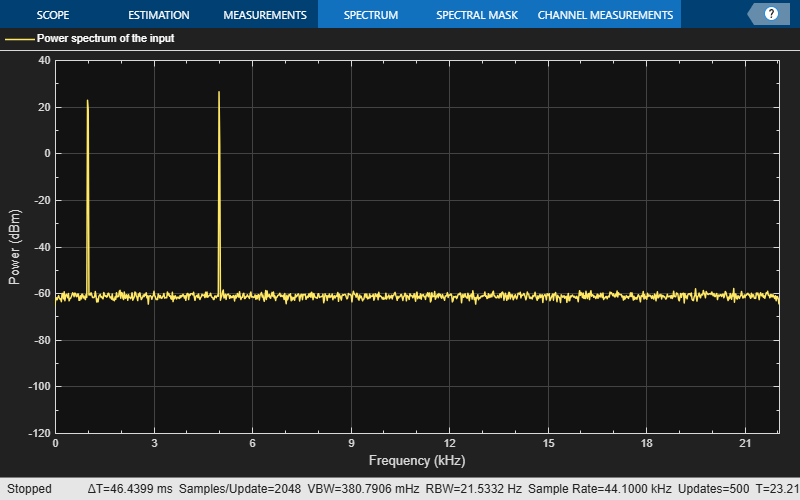In the spectrum analyzer output, you can see two distinct peaks: one at 1000 Hz and the other at 5000 Hz.

Resolution Bandwidth (RBW) is the minimum frequency bandwidth that can be resolved by the spectrum analyzer. By default, the `RBWSource` property of the `spectrumAnalyzer` object is set to `"auto"`. In this mode, RBW is the ratio of the frequency span to 1024. In a two-sided spectrum, this value is $\frac{{F}_{s}}{1024}$, while in a one-sided spectrum, it is $\frac{\frac{{F}_{s}}{2}}{1024}$. The spectrum analyzer in this example shows a one-sided spectrum. Hence, RBW is (44100/2)/1024 or 21.53Hz

Using this value of $RBW$, the number of input samples required to compute one spectral update, ${N}_{samples}$ is given by the following equation: ${N}_{samples}=\frac{{F}_{s}}{RBW}$.

In this example, ${N}_{samples}$ is 44100/21.53 or 2048 samples. You can view this value in the status bar by right-clicking the status bar and selecting Samples/Update.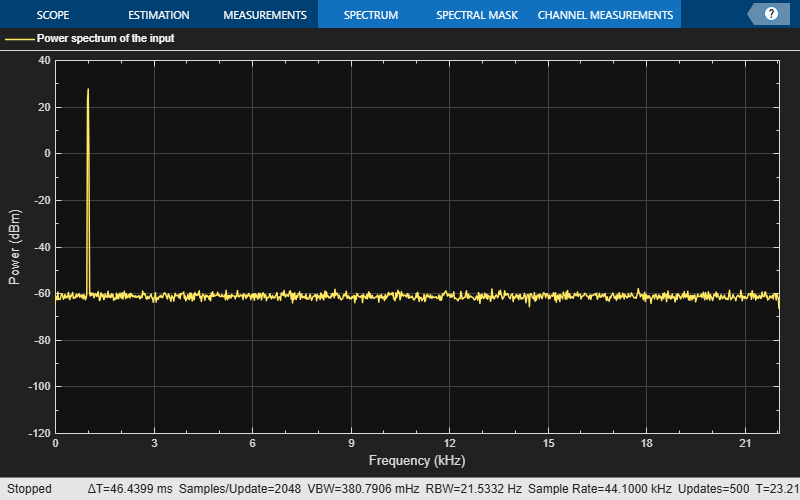$RBW$ calculated in the "auto" mode gives a good frequency resolution.

To distinguish between two frequencies in the display, the distance between the two frequencies must be at least RBW. In this example, the distance between the two peaks is 4000 Hz, which is greater than $RBW$. Hence, you can see the peaks distinctly. Change the frequency of the second sine wave to 1015 Hz. The difference between the two frequencies is less than $RBW$.

```release(Sineobject2); Sineobject2.Frequency = 1015; for Iter = 1:1000 Sinewave1 = Sineobject1(); Sinewave2 = Sineobject2(); Input = Sinewave1 + Sinewave2; NoisyInput = Input + 0.001*randn(1024,1); SA(NoisyInput); end release(SA);```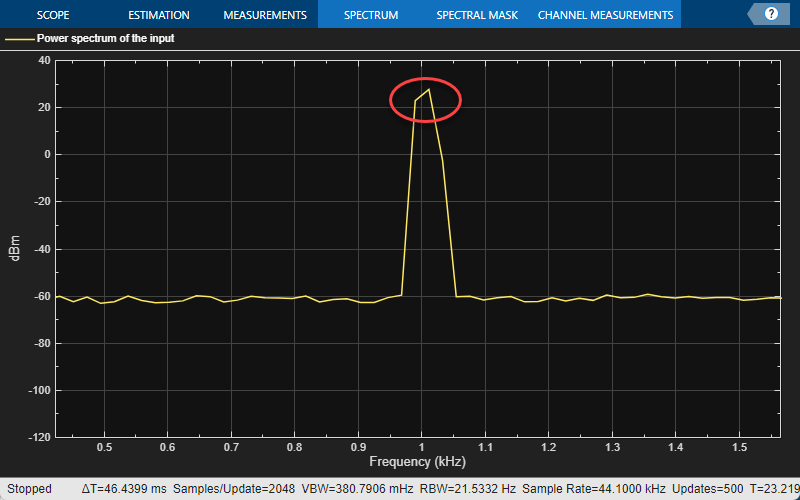The peaks are not distinguishable.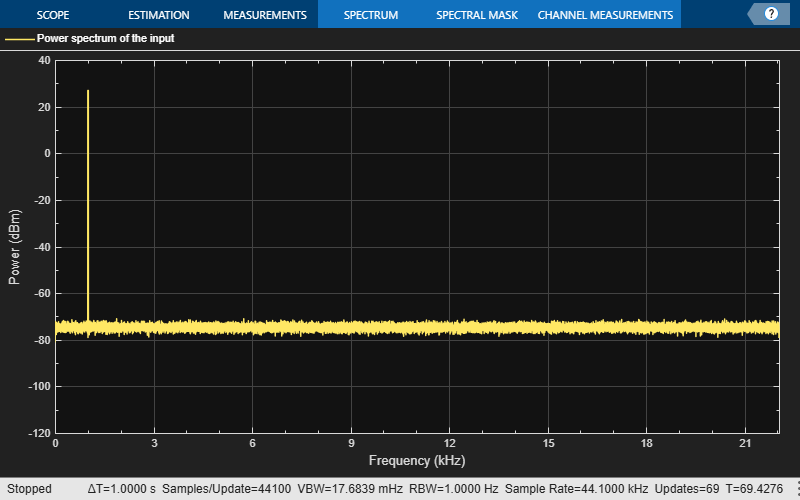To increase the frequency resolution, decrease $RBW$ to 1 Hz.

```SA.RBWSource = "property"; SA.RBW = 1; for Iter = 1:3000 Sinewave1 = Sineobject1(); Sinewave2 = Sineobject2(); Input = Sinewave1 + Sinewave2; NoisyInput = Input + 0.001*randn(1024,1); SA(NoisyInput); end release(SA);```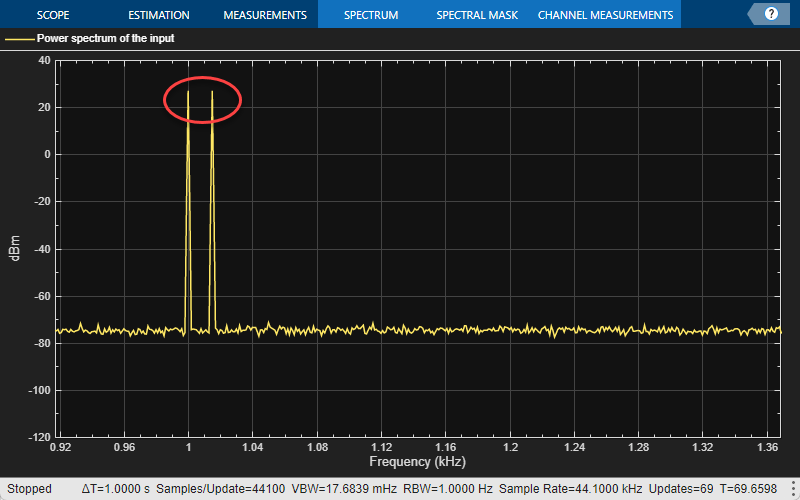On zooming, the two peaks, which are 15 Hz apart, are now distinguishable.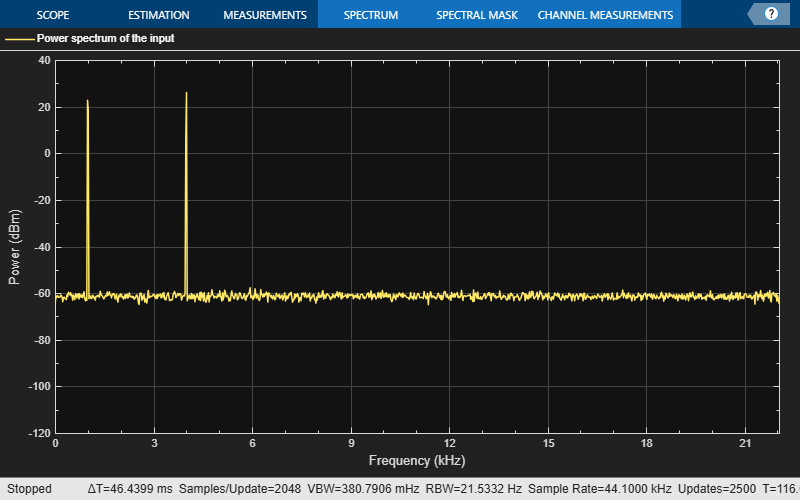When you increase the frequency resolution, the time resolution decreases. To maintain a good balance between the frequency resolution and time resolution, change the `RBWSource` property to `"auto"`.

During streaming, you can change the input properties or the spectrum analyzer properties and see the effect on the spectrum analyzer output immediately. For example, change the frequency of the second sine wave when the index of the loop is a multiple of 1000.

```release(Sineobject2); SA.RBWSource = "auto"; for Iter = 1:5000 Sinewave1 = Sineobject1(); if (mod(Iter,1000) == 0) release(Sineobject2); Sineobject2.Frequency = Iter; Sinewave2 = Sineobject2(); else Sinewave2 = Sineobject2(); end Input = Sinewave1 + Sinewave2; NoisyInput = Input + 0.001*randn(1024,1); SA(NoisyInput); end release(SA);```While running the streaming loop, you can see that the peak of the second sine wave changes according to the iteration value. Similarly, you can change any of the spectrum analyzer properties while the simulation is running and see a corresponding change in the output.

### Convert the Power Between Units

The spectrum analyzer provides three units to specify the power spectral density: `Watts/Hz`, `dBm/Hz`, and `dBW/Hz`. Corresponding units of power are `Watts`, `dBm`, and `dBW`. For electrical engineering applications, you can also view the RMS of your signal in `Vrms` or `dBV`. The default spectrum type is Power in `dBm`.

#### Convert the Power in Watts to dBW and dBm

Power in `dBW` is given by:

`${P}_{\text{dBW}}=10{\mathrm{log}}_{10}\left(\text{power}\text{\hspace{0.17em}}\text{in}\text{\hspace{0.17em}}\text{watt}/1\text{\hspace{0.17em}}\text{watt}\right)$`

Power in `dBm` is given by:

`${P}_{\text{dBm}}=10{\mathrm{log}}_{10}\left(\text{power}\text{\hspace{0.17em}}\text{in}\text{\hspace{0.17em}}\text{watt}/1\text{\hspace{0.17em}}\text{milliwatt}\right)$`

For a sine wave signal with an amplitude of 1 V, the power of a one-sided spectrum in `Watts` is given by:

`$\begin{array}{l}{P}_{\text{Watts}}={A}^{2}/2\\ {P}_{\text{Watts}}=1/2\end{array}$`

Corresponding power in dBm is given by:

`$\begin{array}{l}{P}_{\text{dBm}}=10{\mathrm{log}}_{10}\left(\text{power}\text{\hspace{0.17em}}\text{in}\text{\hspace{0.17em}}\text{watt}/1\text{\hspace{0.17em}}\text{milliwatt}\right)\\ {P}_{\text{dBm}}=10{\mathrm{log}}_{10}\left(0.5/{10}^{-3}\right)\\ {P}_{\text{dBm}}=26.9897\text{\hspace{0.17em}}\text{dBm}\end{array}$`

To confirm this value with a peak finder, enable Peak Finder in the Measurements tab of the Spectrum Analyzer toolstrip.

For a white noise signal, the spectrum is flat for all frequencies. The Spectrum Analyzer in this example shows a one-sided spectrum in the range [0 Fs/2]. For a white noise signal with a variance of 1e-4, the power per unit bandwidth (Punitbandwidth) is 1e-4. The total power of white noise in `Watts` over the entire frequency range is given by:

`$\begin{array}{l}{P}_{\text{whitenoise}}={P}_{\text{unitbandwidth}}.\text{number}\text{\hspace{0.17em}}\text{of}\text{\hspace{0.17em}}\text{frequency}\text{\hspace{0.17em}}\text{bins},\\ {P}_{\text{whitenoise}}=\left({10}^{-4}\right).\left(\frac{Fs/2}{RBW}\right)\end{array}$`

Fs is the input sample rate. The number of frequency bins is the ratio of total bandwidth to RBW. For a one-sided spectrum, the total bandwidth is half the sample rate. With a sample rate of 44100 Hz and a RBW of 21.53 Hz, the total power of white noise in `Watts` is 0.1024 W.

`$\begin{array}{l}{P}_{\text{whitenoise}}=\left({10}^{-4}\right).\left(\frac{22050}{21.53}\right)\\ {P}_{\text{whitenoise}}=0.1024\text{\hspace{0.17em}}\text{W}\end{array}$`

In dBm, the power of white noise can be calculated using:

`$\begin{array}{l}{P}_{\text{whitenoise}}=10{\mathrm{log}}_{10}\left(0.1024/{10}^{-3}\right)\\ {P}_{\text{whitenoise}}=20.103\text{\hspace{0.17em}}\text{dBm}\end{array}$`

#### Convert Power in Watts to dBFS

If you set the spectral units to `dBFS` and set the full scale (`FullScaleSource`) to `"auto"`, power in `dBFS` is computed as:

`${P}_{\text{dBFS}}=20\cdot {\mathrm{log}}_{10}\left(\sqrt{{P}_{\text{watts}}}/\text{Full_Scale}\right)$`

where:

• Pwatts is the power in watts

• For double and float signals, Full_Scale is the maximum value of the input signal.

• For fixed point or integer signals, Full_Scale is the maximum value that can be represented.

If you specify a manual full scale (set `FullScaleSource` to `"property"`), power in `dBFS` is given by:

`${P}_{\text{FS}}=20\cdot {\mathrm{log}}_{10}\left(\sqrt{{P}_{\text{watts}}}/\text{FS}\right)$`

Where `FS` is the full scaling factor specified in the `FullScale` property.

For a sine wave signal with an amplitude of 1 V, the power of a one-sided spectrum in `dBFS` is given by:

`$\begin{array}{l}{P}_{\text{FS}}=20\cdot {\mathrm{log}}_{10}\left(\sqrt{1/2}/1\right)\\ {P}_{\text{FS}}=-3.0103\text{\hspace{0.17em}}\text{dBFS}\end{array}$`

To confirm this value in the Spectrum Analyzer, run these commands:

```Fs = 1000; % Sampling frequency sinef = dsp.SineWave(SampleRate=Fs,SamplesPerFrame=100); scope = spectrumAnalyzer(SampleRate=Fs,... SpectrumUnits="dBFS",PlotAsTwoSidedSpectrum=false) for ii = 1:100000 xsine = sinef(); scope(xsine) end ```
Then, enable Peak Finder in the Measurements tab of the Spectrum Analyzer toolstrip.

#### Convert the Power in dBm to RMS in Vrms

Power in `dBm` is given by:

`${P}_{\text{dBm}}=10{\mathrm{log}}_{10}\left(\text{power}\text{\hspace{0.17em}}\text{in}\text{\hspace{0.17em}}\text{watt}/1\text{\hspace{0.17em}}\text{milliwatt}\right)$`

Voltage in RMS is given by:

`${V}_{\text{rms}}={10}^{{P}_{\text{dBm}}/20}\sqrt{{10}^{-3}}$`

From the previous example, PdBm equals 26.9897 dBm. The Vrms is calculated as

`${V}_{\text{rms}}={10}^{26.9897/20}\sqrt{0.001}$`

which equals 0.7071.

To confirm this value using the peak finder:

1. In the Analyzer Tab of the Spectrum Analyzer toolstrip, select Spectrum > RMS.

2. Enable Peak Finder in the Measurements tab.

### Estimate the Power Spectrum Using dsp.SpectrumEstimator

Alternately, you can compute the power spectrum of the signal using the `dsp.SpectrumEstimator` System object. You can acquire the output of the spectrum estimator and store the data for further processing. To view other objects in the `Estimation` library, type `help dsp` in the MATLAB® command prompt, and click `Estimation`.

Initialization

Use the same source as in the previous section on using the `spectrumAnalyzer` to estimate the power spectrum. The input sine wave has two frequencies: one at 1000 Hz and the other at 5000 Hz. Initialize `dsp.SpectrumEstimator` to compute the power spectrum of the signal using the filter bank approach. View the power spectrum of the signal using the `dsp.ArrayPlot` object.

```Fs = 44100; Sineobject1 = dsp.SineWave(SamplesPerFrame=1024,PhaseOffset=10,... SampleRate=Fs,Frequency=1000); Sineobject2 = dsp.SineWave(SamplesPerFrame=1024,... SampleRate=Fs,Frequency=5000); SpecEst = dsp.SpectrumEstimator(Method='Filter bank',... PowerUnits='dBm',SampleRate=Fs,FrequencyRange='onesided'); ArrPlot = dsp.ArrayPlot(PlotType='Line',ChannelNames={'Power spectrum of the input'},... YLimits=[-80 30],XLabel='Number of samples per frame',YLabel='Power (dBm)',... Title='One-sided power spectrum with respect to samples');```

Estimation

Stream in and estimate the power spectrum of the signal. Construct a `for`-loop to run for 5000 iterations. In each iteration, stream in 1024 samples (one frame) of each sine wave and compute the power spectrum of each frame. Add Gaussian noise with mean at 0 and a standard deviation of 0.001 to the input signal.

```for Iter = 1:5000 Sinewave1 = Sineobject1(); Sinewave2 = Sineobject2(); Input = Sinewave1 + Sinewave2; NoisyInput = Input + 0.001*randn(1024,1); PSoutput = SpecEst(NoisyInput); ArrPlot(PSoutput); end```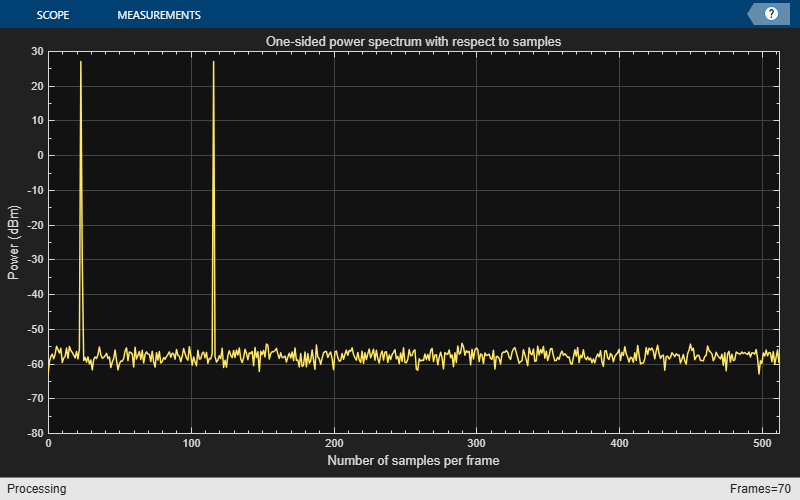Using the filter bank approach, the spectral estimate has a high resolution and the peaks are precise with no spectral leakage.

Convert x-axis to Represent Frequency

By default, the array plot shows the power spectral data with respect to the number of samples per frame. The number of points on the x-axis equals the length of the input frame. The spectrum analyzer plots the power spectral data with respect to frequency. For a one-sided spectrum, the frequency varies in the range [0 Fs/2]. For a two-sided spectrum, the frequency varies in the range [-Fs/2 Fs/2]. To convert the x-axis of the array plot from sample-based to frequency-based, do the following:

• In the Plot tab of the Array Plot toolstrip, click Settings.

• For a one-sided spectrum: Under Data and Axes, set Sample Increment to $Fs/FrameLength$ and X-Offset to 0.

• For a two-sided spectrum: Under Data and Axes, set Sample Increment to $Fs/FrameLength$ and X-Offset to $-Fs/2$.

In this example, the spectrum is one-sided and hence, the Sample Increment and X-Offset are set to 44100/1024 and 0, respectively. To specify the frequency in kHz, set the Sample Increment to 44.1/1024.

```ArrPlot.SampleIncrement = (Fs/1000)/1024; ArrPlot.XLabel = 'Frequency (kHz)'; ArrPlot.Title = 'One-sided power spectrum with respect to frequency'; for Iter = 1:5000 Sinewave1 = Sineobject1(); Sinewave2 = Sineobject2(); Input = Sinewave1 + Sinewave2; NoisyInput = Input + 0.001*randn(1024,1); PSoutput = SpecEst(NoisyInput); ArrPlot(PSoutput); end```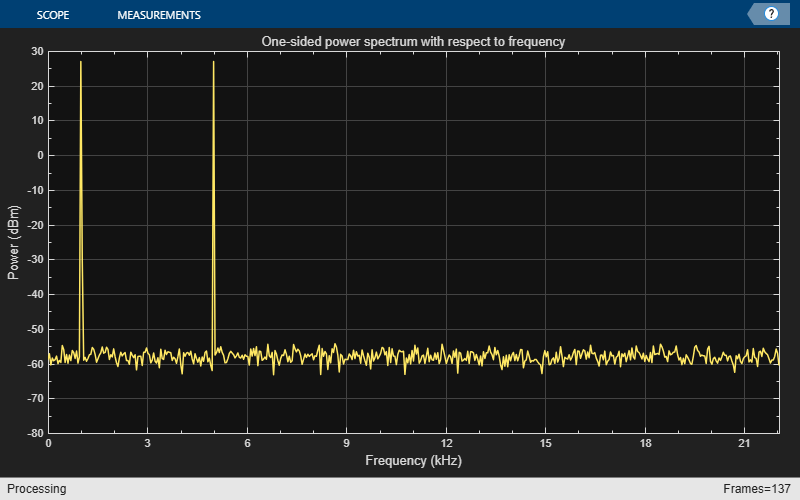Live Processing

The output of the `dsp.SpectrumEstimator` object contains the spectral data and is available for further processing. The data can be processed in real-time or it can be stored in the workspace.